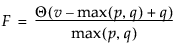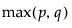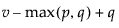Publication date: 11/10/2021

## Approximate F-Tests

To compute F-values and degrees of freedom, let p be the rank of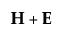. Let q be the rank of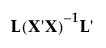, where the L matrix identifies elements of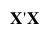associated with the effect being tested. Let v be the error degrees of freedom and s be the minimum of p and q. Also let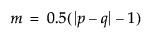and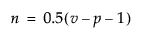.

Table 9.2, gives the computation of each approximate F from the corresponding test statistic.

Table 9.2 Approximate F-statistics

Test

Approximate F

Numerator DF

Denominator DF

Wilks’ Lambda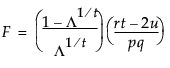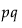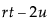Pillai’s Trace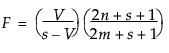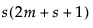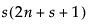Hotelling-Lawley Trace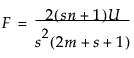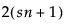Roy’s Max Root